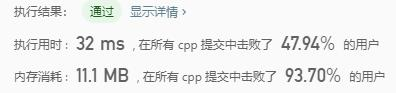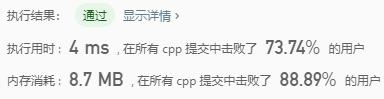# Leetcode 347：前k个高频元素

## 代码实现

vector<int> topKFrequent(vector<int>& nums, int k) { map<int, int> mymap; for (int i = 0; i < nums.size(); i++) { if (mymap.find(nums[i]) != mymap.end())//复杂度O(1) ++mymap[nums[i]]; else { mymap.insert(pair<int, int>(nums[i], 1)); } } vector<int>result; vector<pair<int, int>>mapsort(mymap.begin(), mymap.end()); sort(mapsort.begin(), mapsort.end(), [](pair<int, int>& a, pair<int, int>& b) {return a.second > b.second; }); int count = 0; for (auto it = mapsort.begin(); count < k; count++, ++it) { result.push_back(it->first); } return result;}

## 代码性能# Leetcode 394 字符串解码

## 示例

s = “3[a]2[bc]”, 返回 “aaabcbc”.
s = “3[a2]“, 返回 “accaccacc”.
s = “2[abc]3[cd]ef”, 返回 “abcabccdcdcdef”.

## 代码实现

string decodeString(string s) { string result = ""; stack<int>nums; stack<string>str; int num = 0; int len = s.size(); for (int i = 0; i < len; i++) { if (s[i] >= '0' && s[i] <= '9') { num = num * 10 + s[i] - '0'; } else if ((s[i] >= 'a' && s[i] <= 'z')||(s[i]>='A'&&s[i]<='Z')) { result = result + s[i]; } else if (s[i] == '[')//将‘[’前的数字压入nums栈内， 字母字符串压入strs栈内 { nums.push(num); num = 0; str.push(result); result = ""; } else if (s[i] == ']') //遇到‘]’时，操作与之相配的‘[’之间的字符，使用分配律 { int times = nums.top(); nums.pop(); for (int j = 0; j < times; j++) { str.top() += result; //之后若还是字母，就会直接加到res之后，因为它们是同一级的运算 //若是左括号，res会被压入strs栈，作为上一层的运算 } result = str.top(); str.pop(); } } return result;}

## 复杂度